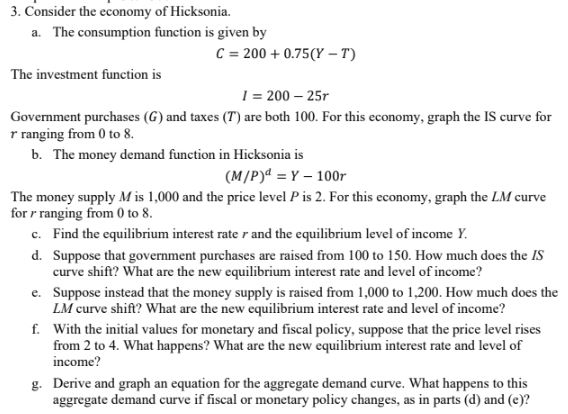Home / Answered Questions / Other / 3-consider-the-economy-of-hicksonia-a-the-consumption-function-is-given-by-c-200-0-75-y-t-the-invest-aw690

# (Solved): 3. Consider The Economy Of Hicksonia. A. The Consumption Function Is Given By C = 200 +0.75(Y-T) The...3. Consider the economy of Hicksonia. a. The consumption function is given by C = 200 +0.75(Y-T) The investment function is 1 = 200 - 251 Government purchases (G) and taxes (T) are both 100. For this economy, graph the IS curve for r ranging from 0 to 8. b. The money demand function in Hicksonia is (M/P) = Y - 100r The money supply M is 1,000 and the price level P is 2. For this economy, graph the LM curve for r ranging from 0 to 8. c. Find the equilibrium interest rater and the equilibrium level of income Y. d. Suppose that government purchases are raised from 100 to 150. How much does the IS curve shift? What are the new equilibrium interest rate and level of income? e. Suppose instead that the money supply is raised from 1,000 to 1,200. How much does the LM curve shift? What are the new equilibrium interest rate and level of income? f. With the initial values for monetary and fiscal policy, suppose that the price level rises from 2 to 4. What happens? What are the new equilibrium interest rate and level of income? g. Derive and graph an equation for the aggregate demand curve. What happens to this aggregate demand curve if fiscal or monetary policy changes, as in parts (d) and (c)?

We have an Answer from Expert